# NCERT Exemplar Solutions for Class 12 Maths Chapter 1 Relations and Functions

The NCERT Exemplar materials are available for various classes, subject-wise. Students referring to these books can grasp the concepts better. In order to help them, the NCERT Exemplar Solutions have been created by subject experts at BYJU’S. These solutions provide exercise problems to questions in all chapters. Also, the solutions are according to the latest CBSE syllabus.

The first chapter of NCERT Exemplar Solutions for Class 12 Maths is Relations and Functions. The chapter deals with different types of relations and functions. Further, the composition of functions, invertible functions and binary operations are discussed. Students who wish to learn easy tricks and shortcuts to solve exercise problems can access the solutions PDF of NCERT Exemplar Solutions for Class 12 Maths Chapter 1 Relations and Functions from the link below.

## Download the PDF of NCERT Exemplar Solutions for Class 12 Maths Chapter 1 Relations and Functions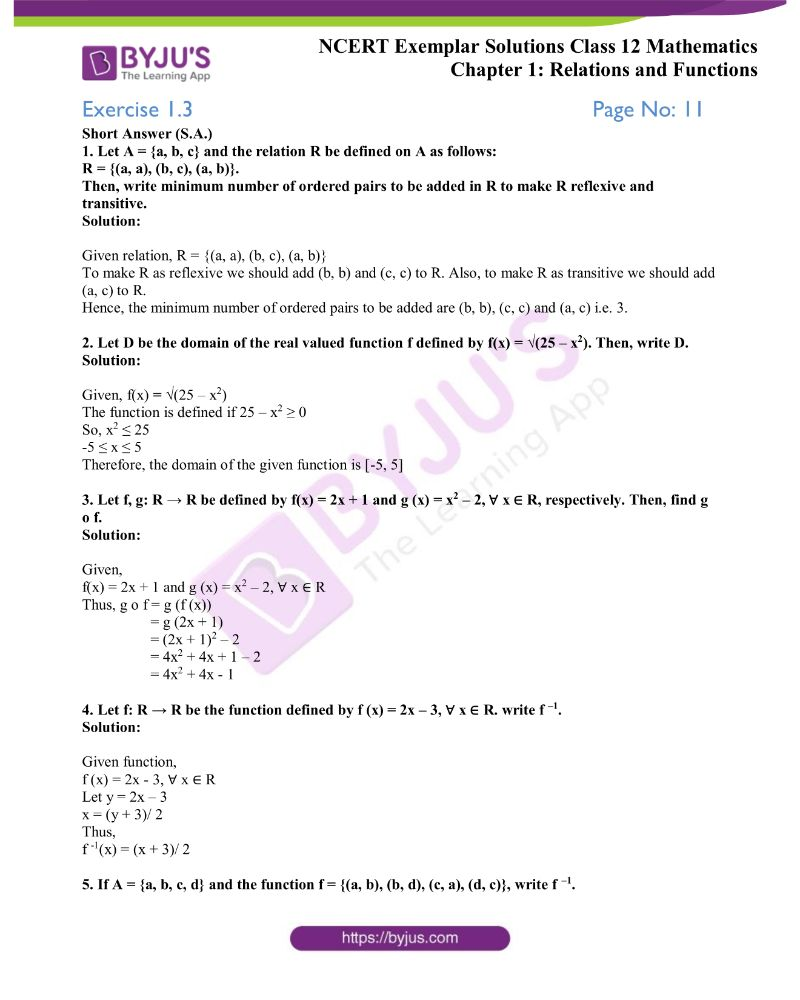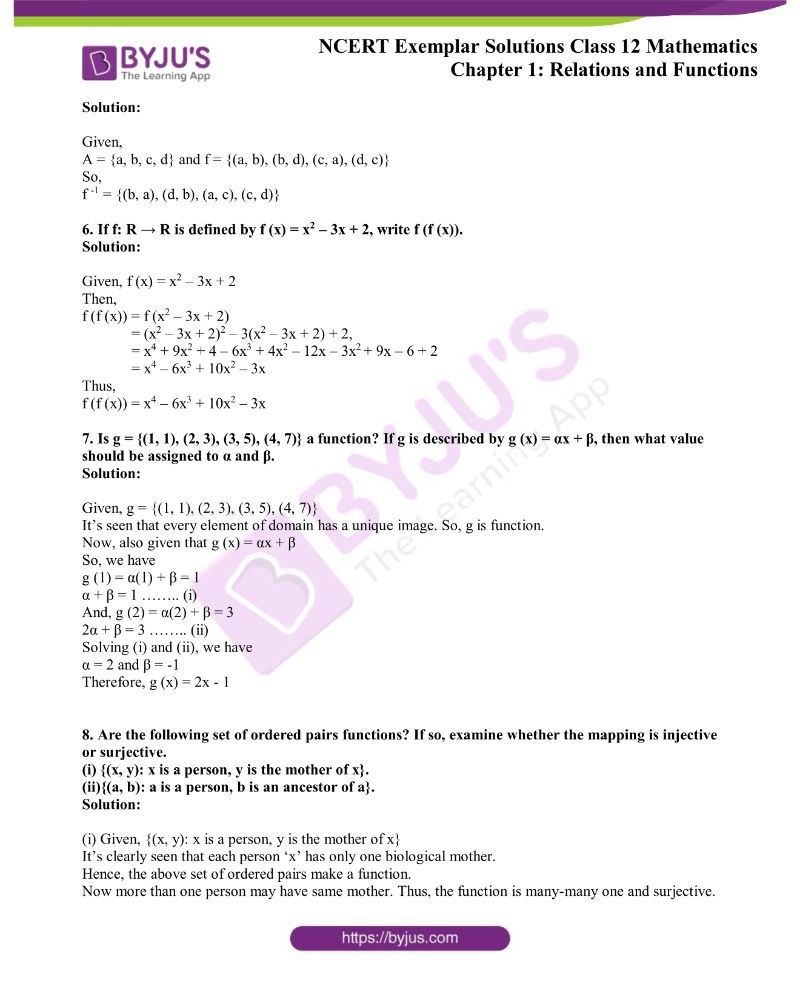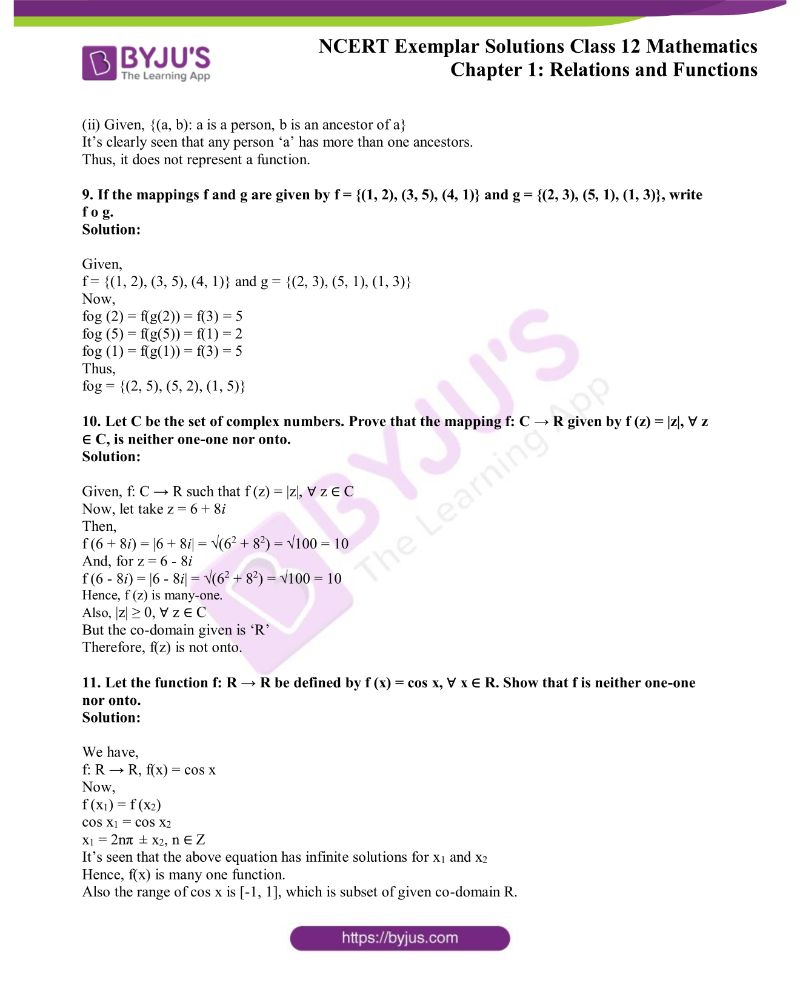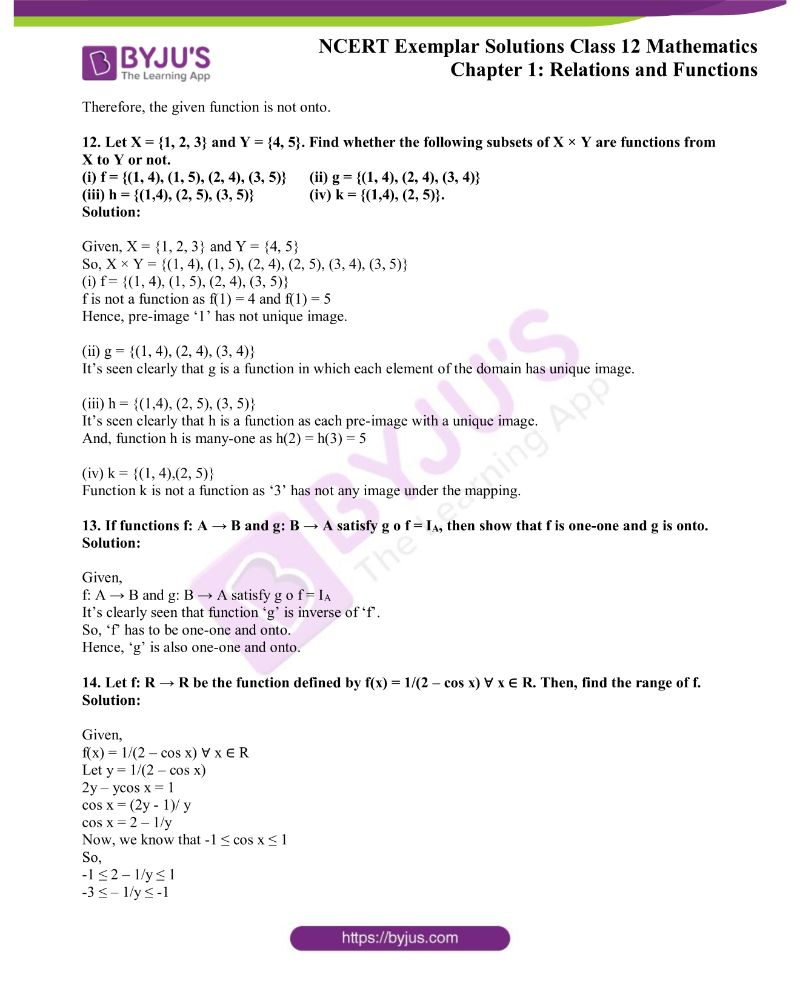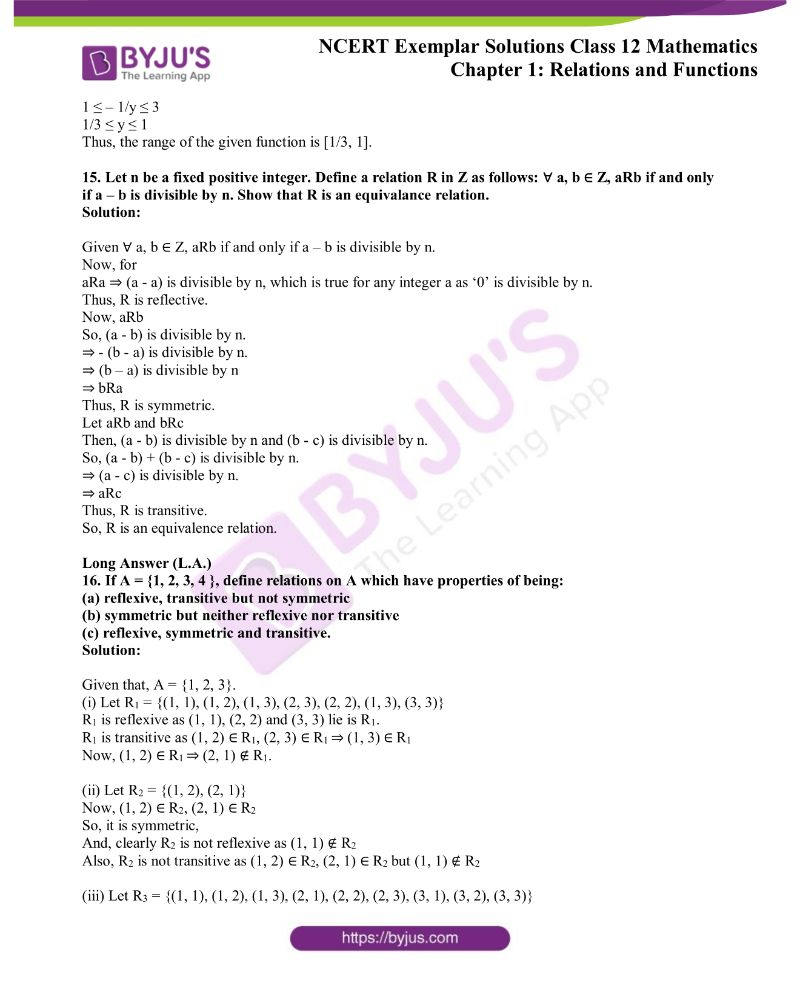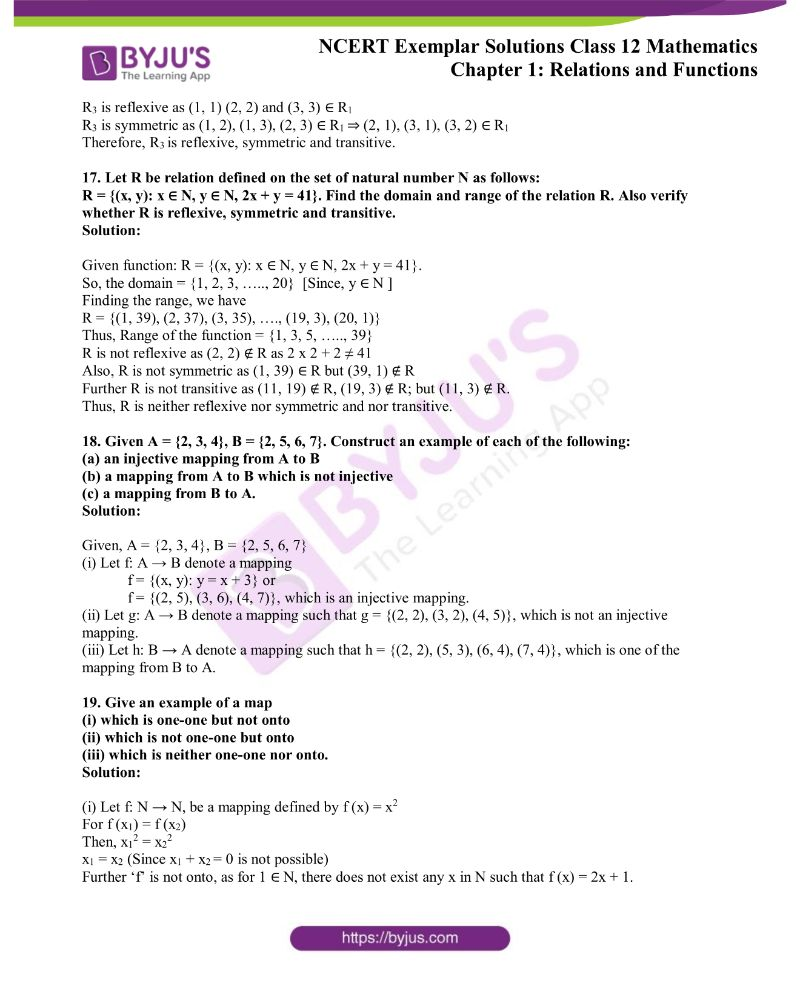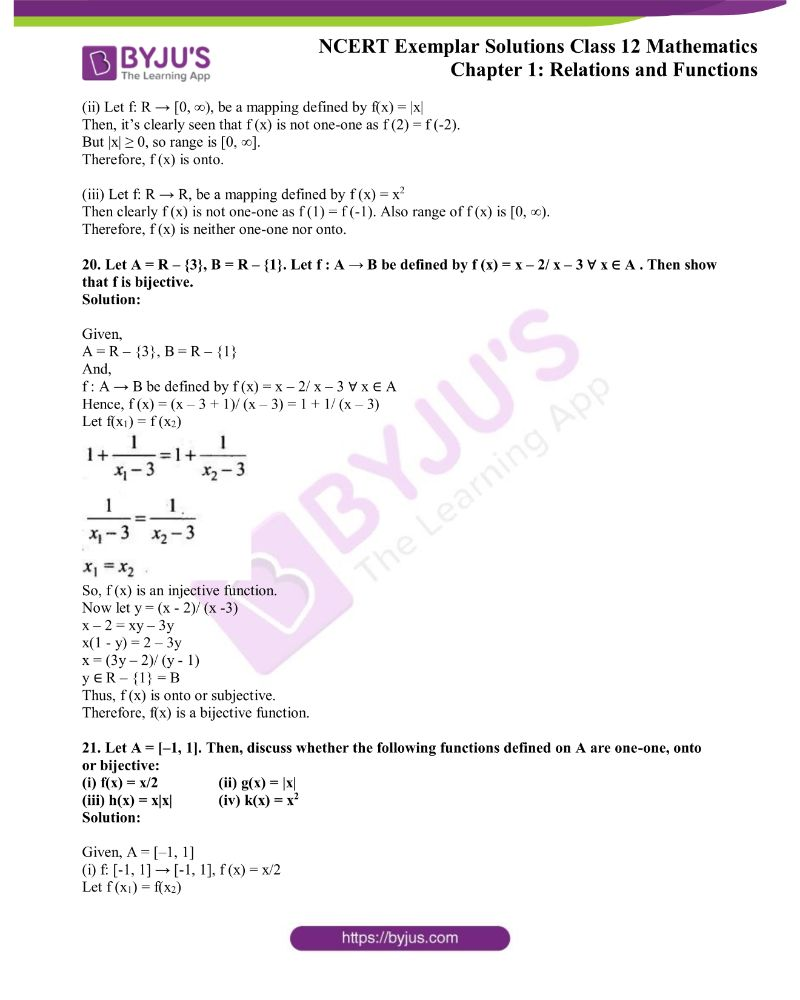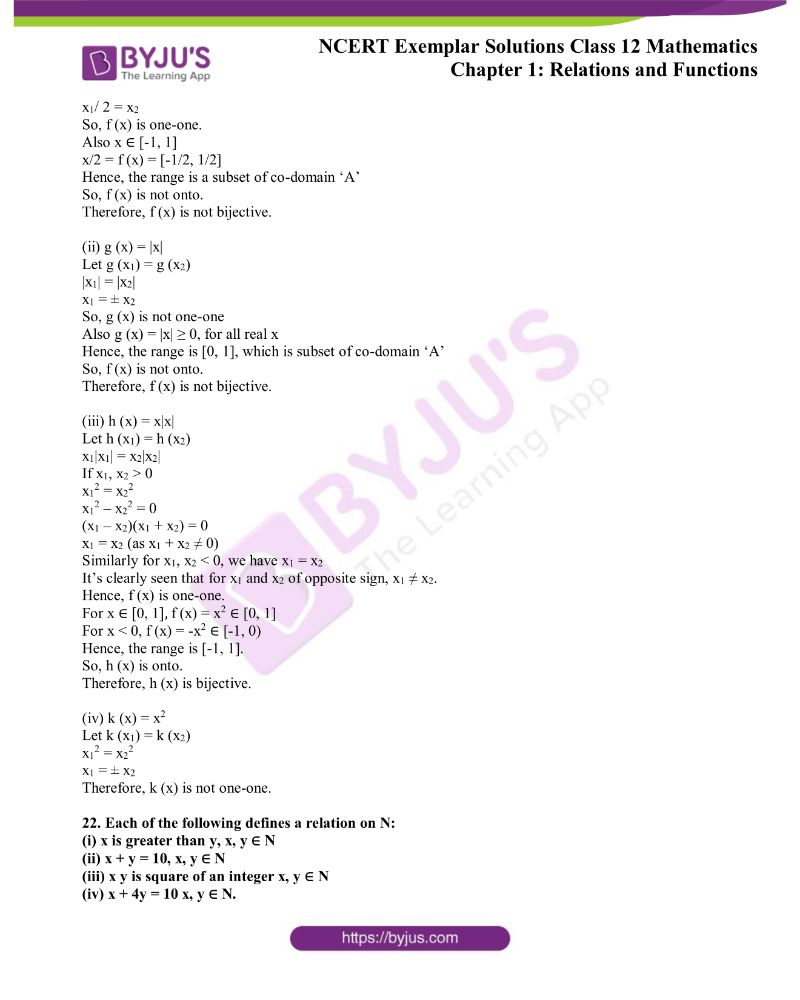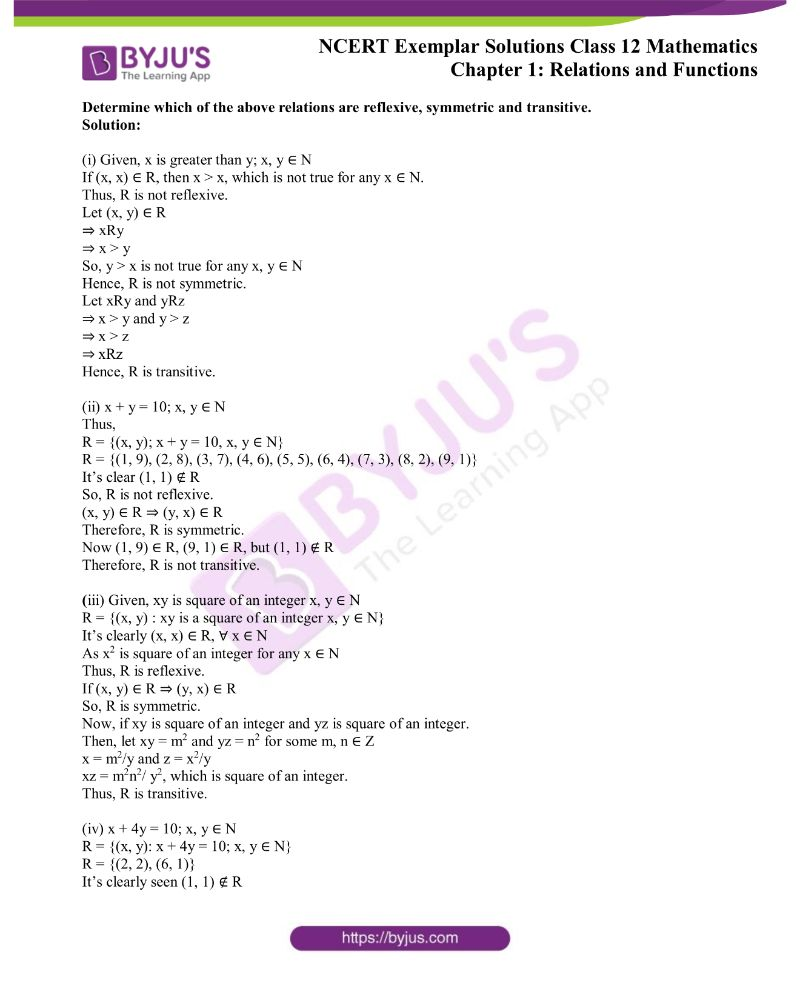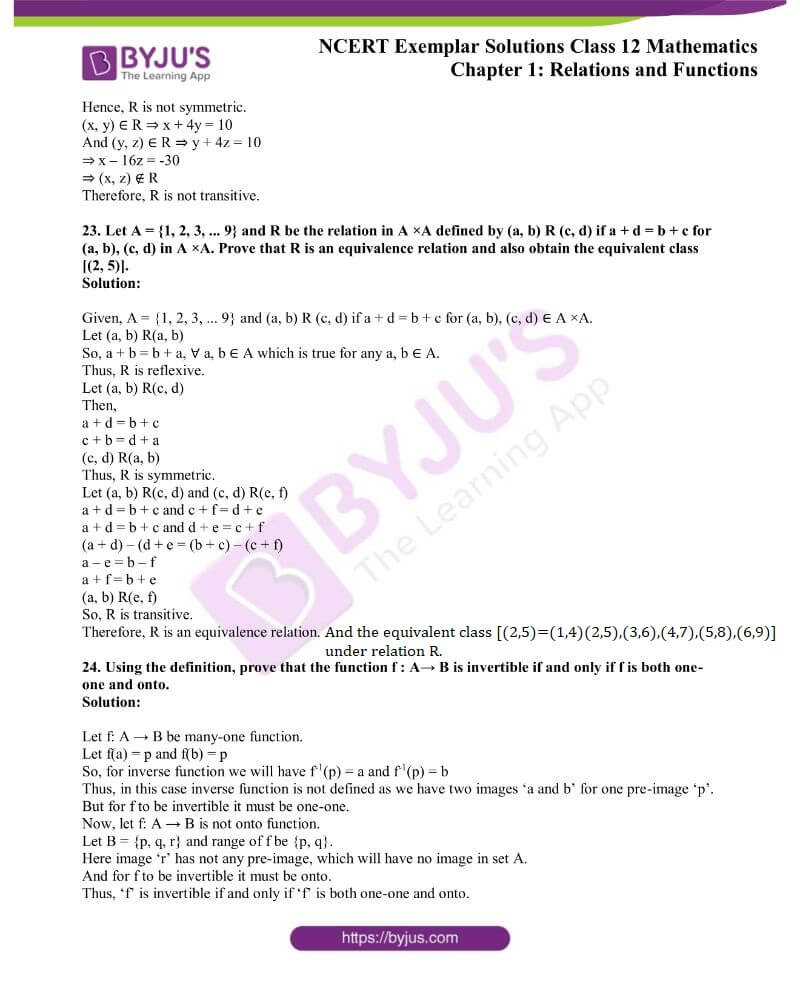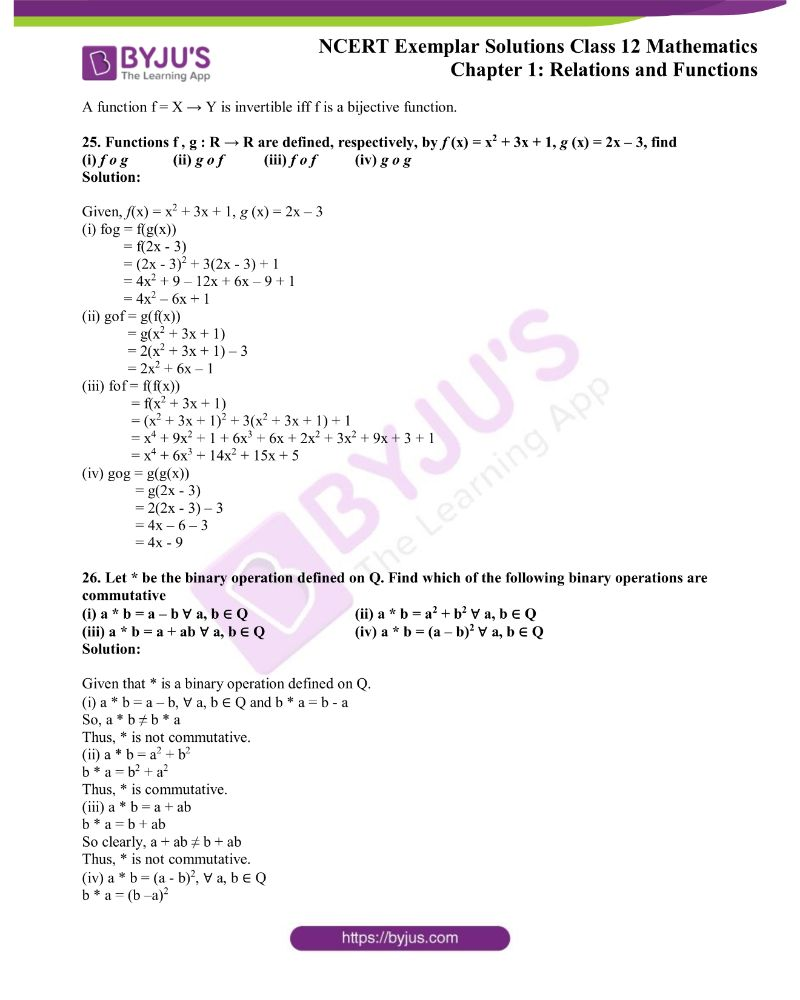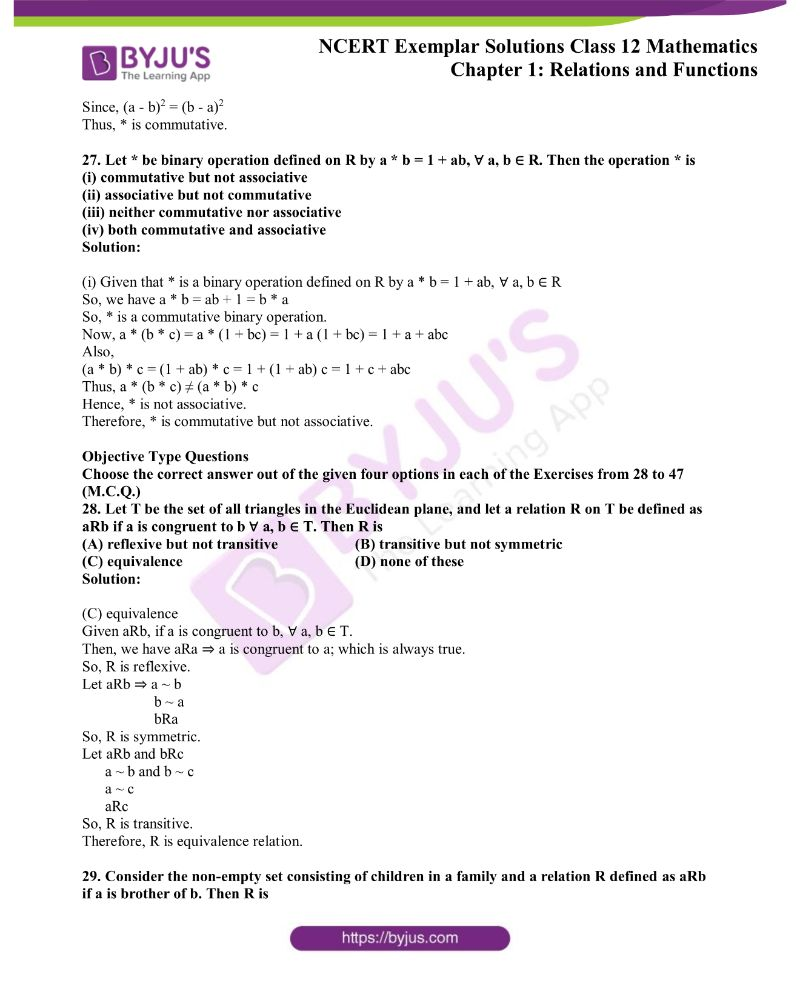### Access Answers to the NCERT Exemplar Class 12 Maths Chapter 1 Relations and Functions

Exercise 1.3 Page No: 11

1. Let A = {a, b, c} and the relation R be defined on A as follows:

R = {(a, a), (b, c), (a, b)}.

Then, write minimum number of ordered pairs to be added in R to make R reflexive and transitive.

Solution:

Given relation, R = {(a, a), (b, c), (a, b)}

To make R as reflexive we should add (b, b) and (c, c) to R. Also, to make R as transitive we should add (a, c) to R.

Hence, the minimum number of ordered pairs to be added are (b, b), (c, c) and (a, c) i.e. 3.

2. Let D be the domain of the real valued function f defined by f(x) = √(25 – x2). Then, write D.

Solution:

Given, f(x) = √(25 – x2)

The function is defined if 25 – x2 ≥ 0

So, x2 ≤ 25

-5 ≤ x ≤ 5

Therefore, the domain of the given function is [-5, 5].

3. Let f, g: R → R be defined by f(x) = 2x + 1 and g (x) = x2 – 2, ∀ x ∈ R, respectively. Then, find g o f.

Solution:

Given,

f(x) = 2x + 1 and g (x) = x2 – 2, ∀ x ∈ R

Thus, g o f = g (f (x))

= g (2x + 1)

= (2x + 1)2 – 2

= 4x2 + 4x + 1 – 2

= 4x2 + 4x – 1

4. Let f: R → R be the function defined by f (x) = 2x – 3, ∀ x ∈ R. write f –1.

Solution:

Given function,

f (x) = 2x – 3, ∀ x ∈ R

Let y = 2x – 3

x = (y + 3)/ 2

Thus,

f -1(x) = (x + 3)/ 2

5. If A = {a, b, c, d} and the function f = {(a, b), (b, d), (c, a), (d, c)}, write f –1.

Solution:

Given,

A = {a, b, c, d} and f = {(a, b), (b, d), (c, a), (d, c)}

So,

f -1 = {(b, a), (d, b), (a, c), (c, d)}

6. If f: R → R is defined by f (x) = x2 – 3x + 2, write f (f (x)).

Solution:

Given, f (x) = x2 – 3x + 2

Then,

f (f (x)) = f (x2 – 3x + 2)

= (x2 – 3x + 2)2 – 3(x2 – 3x + 2) + 2,

= x4 + 9x2 + 4 – 6x3 + 4x2 – 12x – 3x+ 9x – 6 + 2

= x4 – 6x3 + 10x2 – 3x

Thus,

f (f (x)) = x4 – 6x3 + 10x2 – 3x

7. Is g = {(1, 1), (2, 3), (3, 5), (4, 7)} a function? If g is described by g (x) = αx + β, then what value should be assigned to α and β.

Solution:

Given, g = {(1, 1), (2, 3), (3, 5), (4, 7)}

It’s seen that every element of domain has a unique image. So, g is function.

Now, also given that g (x) = αx + β

So, we have

g (1) = α(1) + β = 1

α + β = 1 …….. (i)

And, g (2) = α(2) + β = 3

2α + β = 3 …….. (ii)

Solving (i) and (ii), we have

α = 2 and β = -1

Therefore, g (x) = 2x – 1

8. Are the following set of ordered pairs functions? If so, examine whether the mapping is injective or surjective.

(i) {(x, y): x is a person, y is the mother of x}.

(ii){(a, b): a is a person, b is an ancestor of a}.

Solution:

(i) Given, {(x, y): x is a person, y is the mother of x}

It’s clearly seen that each person ‘x’ has only one biological mother.

Hence, the above set of ordered pairs make a function.

Now more than one person may have same mother. Thus, the function is many-many one and surjective.

(ii) Given, {(a, b): a is a person, b is an ancestor of a}

It’s clearly seen that any person ‘a’ has more than one ancestors.

Thus, it does not represent a function.

9. If the mappings f and g are given by f = {(1, 2), (3, 5), (4, 1)} and g = {(2, 3), (5, 1), (1, 3)}, write f o g.

Solution:

Given,

f = {(1, 2), (3, 5), (4, 1)} and g = {(2, 3), (5, 1), (1, 3)}

Now,

fog (2) = f(g(2)) = f(3) = 5

fog (5) = f(g(5)) = f(1) = 2

fog (1) = f(g(1)) = f(3) = 5

Thus,

fog = {(2, 5), (5, 2), (1, 5)}

10. Let C be the set of complex numbers. Prove that the mapping f: C → R given by f (z) = |z|, ∀ z ∈ C, is neither one-one nor onto.

Solution:

Given, f: C → R such that f (z) = |z|, ∀ z ∈ C

Now, let take z = 6 + 8i

Then,

f (6 + 8i) = |6 + 8i| = √(62 + 82) = √100 = 10

And, for z = 6 – 8i

f (6 – 8i) = |6 – 8i| = √(62 + 82) = √100 = 10

Hence, f (z) is many-one.

Also, |z| ≥ 0, ∀ z ∈ C

But the co-domain given is ‘R’

Therefore, f(z) is not onto.

11. Let the function f: R → R be defined by f (x) = cos x, ∀ x ∈ R. Show that f is neither one-one nor onto.

Solution:

We have,

f: R → R, f(x) = cos x

Now,

f (x1) = f (x2)

cos x1 = cos x2

x1 = 2nπ ± x2, n ∈ Z

It’s seen that the above equation has infinite solutions for x1 and x2

Hence, f(x) is many one function.

Also the range of cos x is [-1, 1], which is subset of given co-domain R.

Therefore, the given function is not onto.

12. Let X = {1, 2, 3} and Y = {4, 5}. Find whether the following subsets of X × Y are functions from X to Y or not.

(i) f = {(1, 4), (1, 5), (2, 4), (3, 5)} (ii) g = {(1, 4), (2, 4), (3, 4)}

(iii) h = {(1,4), (2, 5), (3, 5)} (iv) k = {(1,4), (2, 5)}.

Solution:

Given, X = {1, 2, 3} and Y = {4, 5}

So, X × Y = {(1, 4), (1, 5), (2, 4), (2, 5), (3, 4), (3, 5)}

(i) f = {(1, 4), (1, 5), (2, 4), (3, 5)}

f is not a function as f(1) = 4 and f(1) = 5

Hence, pre-image ‘1’ has not unique image.

(ii) g = {(1, 4), (2, 4), (3, 4)}

It’s seen clearly that g is a function in which each element of the domain has unique image.

(iii) h = {(1,4), (2, 5), (3, 5)}

It’s seen clearly that h is a function as each pre-image with a unique image.

And, function h is many-one as h(2) = h(3) = 5

(iv) k = {(1, 4),(2, 5)}

Function k is not a function as ‘3’ has not any image under the mapping.

13. If functions f: A → B and g: B → A satisfy g o f = IA, then show that f is one-one and g is onto.

Solution:

Given,

f: A → B and g: B → A satisfy g o f = IA

It’s clearly seen that function ‘g’ is inverse of ‘f’.

So, ‘f’ has to be one-one and onto.

Hence, ‘g’ is also one-one and onto.

14. Let f: R → R be the function defined by f(x) = 1/(2 – cos x) ∀ x ∈ R. Then, find the range of f.

Solution:

Given,

f(x) = 1/(2 – cos x) ∀ x ∈ R

Let y = 1/(2 – cos x)

2y – ycos x = 1

cos x = (2y – 1)/ y

cos x = 2 – 1/y

Now, we know that -1 ≤ cos x ≤ 1

So,

-1 ≤ 2 – 1/y ≤ 1

-3 ≤ – 1/y ≤ -1

1 ≤ – 1/y ≤ 3

1/3 ≤ y ≤ 1

Thus, the range of the given function is [1/3, 1].

15. Let n be a fixed positive integer. Define a relation R in Z as follows: ∀ a, b ∈ Z, aRb if and only if a – b is divisible by n. Show that R is an equivalance relation.

Solution:

Given ∀ a, b ∈ Z, aRb if and only if a – b is divisible by n.

Now, for

aRa ⇒ (a – a) is divisible by n, which is true for any integer a as ‘0’ is divisible by n.

Thus, R is reflective.

Now, aRb

So, (a – b) is divisible by n.

⇒ – (b – a) is divisible by n.

⇒ (b – a) is divisible by n

⇒ bRa

Thus, R is symmetric.

Let aRb and bRc

Then, (a – b) is divisible by n and (b – c) is divisible by n.

So, (a – b) + (b – c) is divisible by n.

⇒ (a – c) is divisible by n.

⇒ aRc

Thus, R is transitive.

So, R is an equivalence relation.

16. If A = {1, 2, 3, 4 }, define relations on A which have properties of being:

(a) reflexive, transitive but not symmetric

(b) symmetric but neither reflexive nor transitive

(c) reflexive, symmetric and transitive.

Solution:

Given that, A = {1, 2, 3}.

(i) Let R1 = {(1, 1), (1, 2), (1, 3), (2, 3), (2, 2), (1, 3), (3, 3)}

R1 is reflexive as (1, 1), (2, 2) and (3, 3) lie is R1.

R1 is transitive as (1, 2) ∈ R1, (2, 3) ∈ R1 ⇒ (1, 3) ∈ R1

Now, (1, 2) ∈ R1 ⇒ (2, 1) ∉ R1.

(ii) Let R2 = {(1, 2), (2, 1)}

Now, (1, 2) ∈ R2, (2, 1) ∈ R2

So, it is symmetric,

And, clearly R2 is not reflexive as (1, 1) ∉ R2

Also, R2 is not transitive as (1, 2) ∈ R2, (2, 1) ∈ R2 but (1, 1) ∉ R2

(iii) Let R3 = {(1, 1), (1, 2), (1, 3), (2, 1), (2, 2), (2, 3), (3, 1), (3, 2), (3, 3)}

R3 is reflexive as (1, 1) (2, 2) and (3, 3) ∈ R1

R3 is symmetric as (1, 2), (1, 3), (2, 3) ∈ R1 ⇒ (2, 1), (3, 1), (3, 2) ∈ R1

Therefore, R3 is reflexive, symmetric and transitive.

17. Let R be relation defined on the set of natural number N as follows:

R = {(x, y): x ∈ N, y ∈ N, 2x + y = 41}. Find the domain and range of the relation R. Also verify whether R is reflexive, symmetric and transitive.

Solution:

Given function: R = {(x, y): x ∈ N, y ∈ N, 2x + y = 41}.

So, the domain = {1, 2, 3, ….., 20} [Since, y ∈ N ]

Finding the range, we have

R = {(1, 39), (2, 37), (3, 35), …., (19, 3), (20, 1)}

Thus, Range of the function = {1, 3, 5, ….., 39}

R is not reflexive as (2, 2) ∉ R as 2 x 2 + 2 ≠ 41

Also, R is not symmetric as (1, 39) ∈ R but (39, 1) ∉ R

Further R is not transitive as (11, 19) ∉ R, (19, 3) ∉ R; but (11, 3) ∉ R.

Thus, R is neither reflexive nor symmetric and nor transitive.

18. Given A = {2, 3, 4}, B = {2, 5, 6, 7}. Construct an example of each of the following:

(a) an injective mapping from A to B

(b) a mapping from A to B which is not injective

(c) a mapping from B to A.

Solution:

Given, A = {2, 3, 4}, B = {2, 5, 6, 7}

(i) Let f: A → B denote a mapping

f = {(x, y): y = x + 3} or

f = {(2, 5), (3, 6), (4, 7)}, which is an injective mapping.

(ii) Let g: A → B denote a mapping such that g = {(2, 2), (3, 2), (4, 5)}, which is not an injective mapping.

(iii) Let h: B → A denote a mapping such that h = {(2, 2), (5, 3), (6, 4), (7, 4)}, which is one of the mapping from B to A.

19. Give an example of a map

(i) which is one-one but not onto

(ii) which is not one-one but onto

(iii) which is neither one-one nor onto.

Solution:

(i) Let f: N → N, be a mapping defined by f (x) = x2

For f (x1) = f (x2)

Then, x12 = x22

x1 = x2 (Since x1 + x2 = 0 is not possible)

Further ‘f’ is not onto, as for 1 ∈ N, there does not exist any x in N such that f (x) = 2x + 1.

(ii) Let f: R → [0, ∞), be a mapping defined by f(x) = |x|

Then, it’s clearly seen that f (x) is not one-one as f (2) = f (-2).

But |x| ≥ 0, so range is [0, ∞].

Therefore, f (x) is onto.

(iii) Let f: R → R, be a mapping defined by f (x) = x2

Then clearly f (x) is not one-one as f (1) = f (-1). Also range of f (x) is [0, ∞).

Therefore, f (x) is neither one-one nor onto.

20. Let A = R – {3}, B = R – {1}. Let f : A → B be defined by f (x) = x – 2/ x – 3 ∀ x ∈ A . Then show that f is bijective.

Solution:

Given,

A = R – {3}, B = R – {1}

And,

f : A → B be defined by f (x) = x – 2/ x – 3 ∀ x ∈ A

Hence, f (x) = (x – 3 + 1)/ (x – 3) = 1 + 1/ (x – 3)

Let f(x1) = f (x2)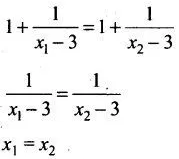So, f (x) is an injective function.

Now let y = (x – 2)/ (x -3)

x – 2 = xy – 3y

x(1 – y) = 2 – 3y

x = (3y – 2)/ (y – 1)

y ∈ R – {1} = B

Thus, f (x) is onto or subjective.

Therefore, f(x) is a bijective function.

21. Let A = [–1, 1]. Then, discuss whether the following functions defined on A are one-one, onto or bijective:

(i) f(x) = x/2 (ii) g(x) = |x|

(iii) h(x) = x|x| (iv) k(x) = x2

Solution:

Given, A = [–1, 1]

(i) f: [-1, 1] → [-1, 1], f (x) = x/2

Let f (x1) = f(x2)

x1/ 2 = x2

So, f (x) is one-one.

Also x ∈ [-1, 1]

x/2 = f (x) = [-1/2, 1/2]

Hence, the range is a subset of co-domain ‘A’

So, f (x) is not onto.

Therefore, f (x) is not bijective.

(ii) g (x) = |x|

Let g (x1) = g (x2)

|x1| = |x2|

x1 = ± x2

So, g (x) is not one-one

Also g (x) = |x| ≥ 0, for all real x

Hence, the range is [0, 1], which is subset of co-domain ‘A’

So, f (x) is not onto.

Therefore, f (x) is not bijective.

(iii) h (x) = x|x|

Let h (x1) = h (x2)

x1|x1| = x2|x2|

If x1, x2 > 0

x12 = x22

x12 – x22 = 0

(x1 – x2)(x1 + x2) = 0

x1 = x2 (as x1 + x2 ≠ 0)

Similarly for x1, x2 < 0, we have x1 = x2

It’s clearly seen that for x1 and x2 of opposite sign, x1 ≠ x2.

Hence, f (x) is one-one.

For x ∈ [0, 1], f (x) = x2 ∈ [0, 1]

For x < 0, f (x) = -x2 ∈ [-1, 0)

Hence, the range is [-1, 1].

So, h (x) is onto.

Therefore, h (x) is bijective.

(iv) k (x) = x2

Let k (x1) = k (x2)

x12 = x22

x1 = ± x2

Therefore, k (x) is not one-one.

22. Each of the following defines a relation on N:

(i) x is greater than y, x, y ∈ N

(ii) x + y = 10, x, y ∈ N

(iii) x y is square of an integer x, y ∈ N

(iv) x + 4y = 10 x, y ∈ N.

Determine which of the above relations are reflexive, symmetric and transitive.

Solution:

(i) Given, x is greater than y; x, y ∈ N

If (x, x) ∈ R, then x > x, which is not true for any x ∈ N.

Thus, R is not reflexive.

Let (x, y) ∈ R

⇒ xRy

⇒ x > y

So, y > x is not true for any x, y ∈ N

Hence, R is not symmetric.

Let xRy and yRz

⇒ x > y and y > z

⇒ x > z

⇒ xRz

Hence, R is transitive.

(ii) x + y = 10; x, y ∈ N

Thus,

R = {(x, y); x + y = 10, x, y ∈ N}

R = {(1, 9), (2, 8), (3, 7), (4, 6), (5, 5), (6, 4), (7, 3), (8, 2), (9, 1)}

It’s clear (1, 1) ∉ R

So, R is not reflexive.

(x, y) ∈ R ⇒ (y, x) ∈ R

Therefore, R is symmetric.

Now (1, 9) ∈ R, (9, 1) ∈ R, but (1, 1) ∉ R

Therefore, R is not transitive.

(iii) Given, xy is square of an integer x, y ∈ N

R = {(x, y) : xy is a square of an integer x, y ∈ N}

It’s clearly (x, x) ∈ R, ∀ x ∈ N

As x2 is square of an integer for any x ∈ N

Thus, R is reflexive.

If (x, y) ∈ R ⇒ (y, x) ∈ R

So, R is symmetric.

Now, if xy is square of an integer and yz is square of an integer.

Then, let xy = m2 and yz = n2 for some m, n ∈ Z

x = m2/y and z = x2/y

xz = m2n2/ y2, which is square of an integer.

Thus, R is transitive.

(iv) x + 4y = 10; x, y ∈ N

R = {(x, y): x + 4y = 10; x, y ∈ N}

R = {(2, 2), (6, 1)}

It’s clearly seen (1, 1) ∉ R

Hence, R is not symmetric.

(x, y) ∈ R ⇒ x + 4y = 10

And (y, z) ∈ R ⇒ y + 4z = 10

⇒ x – 16z = -30

⇒ (x, z) ∉ R

Therefore, R is not transitive.

23. Let A = {1, 2, 3, … 9} and R be the relation in A ×A defined by (a, b) R (c, d) if a + d = b + c for (a, b), (c, d) in A ×A. Prove that R is an equivalence relation and also obtain the equivalent class [(2, 5)].

Solution:

Given, A = {1, 2, 3, … 9} and (a, b) R (c, d) if a + d = b + c for (a, b), (c, d) ∈ A ×A.

Let (a, b) R(a, b)

So, a + b = b + a, ∀ a, b ∈ A which is true for any a, b ∈ A.

Thus, R is reflexive.

Let (a, b) R(c, d)

Then,

a + d = b + c

c + b = d + a

(c, d) R(a, b)

Thus, R is symmetric.

Let (a, b) R(c, d) and (c, d) R(e, f)

a + d = b + c and c + f = d + e

a + d = b + c and d + e = c + f

(a + d) – (d + e = (b + c) – (c + f)

a – e = b – f

a + f = b + e

(a, b) R(e, f)

So, R is transitive.

Therefore, R is an equivalence relation.

And, [(2,5)=(1,4)(2,5),(3,6),(4,7),(5,8),(6,9)] is the equivalent class under relation R.

24. Using the definition, prove that the function f : A→ B is invertible if and only if f is both one-one and onto.

Solution:

Let f: A → B be many-one function.

Let f(a) = p and f(b) = p

So, for inverse function we will have f-1(p) = a and f-1(p) = b

Thus, in this case inverse function is not defined as we have two images ‘a and b’ for one pre-image ‘p’.

But for f to be invertible it must be one-one.

Now, let f: A → B is not onto function.

Let B = {p, q, r} and range of f be {p, q}.

Here image ‘r’ has not any pre-image, which will have no image in set A.

And for f to be invertible it must be onto.

Thus, ‘f’ is invertible if and only if ‘f’ is both one-one and onto.

A function f = X → Y is invertible iff f is a bijective function.

25. Functions f , g : R → R are defined, respectively, by f (x) = x2 + 3x + 1, g (x) = 2x – 3, find

(i) f o g (ii) g o f (iii) f o f (iv) g o g

Solution:

Given, f(x) = x2 + 3x + 1, g (x) = 2x – 3

(i) fog = f(g(x))

= f(2x – 3)

= (2x – 3)2 + 3(2x – 3) + 1

= 4x2 + 9 – 12x + 6x – 9 + 1

= 4x2 – 6x + 1

(ii) gof = g(f(x))

= g(x2 + 3x + 1)

= 2(x2 + 3x + 1) – 3

= 2x2 + 6x – 1

(iii) fof = f(f(x))

= f(x2 + 3x + 1)

= (x2 + 3x + 1)2 + 3(x2 + 3x + 1) + 1

= x4 + 9x2 + 1 + 6x3 + 6x + 2x2 + 3x2 + 9x + 3 + 1

= x4 + 6x3 + 14x2 + 15x + 5

(iv) gog = g(g(x))

= g(2x – 3)

= 2(2x – 3) – 3

= 4x – 6 – 3

= 4x – 9

26. Let * be the binary operation defined on Q. Find which of the following binary operations are commutative

(i) a * b = a – b ∀ a, b ∈Q (ii) a * b = a2 + b2 ∀ a, b ∈ Q

(iii) a * b = a + ab ∀ a, b ∈ Q (iv) a * b = (a – b)2 ∀ a, b ∈ Q

Solution:

Given that * is a binary operation defined on Q.

(i) a * b = a – b, ∀ a, b ∈Q and b * a = b – a

So, a * b ≠ b * a

Thus, * is not commutative.

(ii) a * b = a2 + b2

b * a = b2 + a2

Thus, * is commutative.

(iii) a * b = a + ab

b * a = b + ab

So clearly, a + ab ≠ b + ab

Thus, * is not commutative.

(iv) a * b = (a – b)2, ∀ a, b ∈Q

b * a = (b –a)2

Since, (a – b)2 = (b – a)2

Thus, * is commutative.

27. Let * be binary operation defined on R by a * b = 1 + ab, ∀ a, b ∈ R. Then the operation * is

(i) commutative but not associative

(ii) associative but not commutative

(iii) neither commutative nor associative

(iv) both commutative and associative

Solution:

(i) Given that * is a binary operation defined on R by a * b = 1 + ab, ∀ a, b ∈ R

So, we have a * b = ab + 1 = b * a

So, * is a commutative binary operation.

Now, a * (b * c) = a * (1 + bc) = 1 + a (1 + bc) = 1 + a + abc

Also,

(a * b) * c = (1 + ab) * c = 1 + (1 + ab) c = 1 + c + abc

Thus, a * (b * c) ≠ (a * b) * c

Hence, * is not associative.

Therefore, * is commutative but not associative.

Objective Type Questions

Choose the correct answer out of the given four options in each of the Exercises from 28 to 47 (M.C.Q.)

28. Let T be the set of all triangles in the Euclidean plane, and let a relation R on T be defined as aRb if a is congruent to b ∀ a, b ∈ T. Then R is

(A) reflexive but not transitive (B) transitive but not symmetric

(C) equivalence (D) none of these

Solution:

(C) equivalence

Given aRb, if a is congruent to b, ∀ a, b ∈ T.

Then, we have aRa ⇒ a is congruent to a; which is always true.

So, R is reflexive.

Let aRb ⇒ a ~ b

b ~ a

bRa

So, R is symmetric.

Let aRb and bRc

a ~ b and b ~ c

a ~ c

aRc

So, R is transitive.

Therefore, R is equivalence relation.

29. Consider the non-empty set consisting of children in a family and a relation R defined as aRb if a is brother of b. Then R is

(A) symmetric but not transitive (B) transitive but not symmetric

(C) neither symmetric nor transitive (D) both symmetric and transitive

Solution:

(B) transitive but not symmetric

aRb ⇒ a is brother of b.

This does not mean b is also a brother of a as b can be a sister of a.

Thus, R is not symmetric.

aRb ⇒ a is brother of b.

and bRc ⇒ b is brother of c.

So, a is brother of c.

Therefore, R is transitive.

30. The maximum number of equivalence relations on the set A = {1, 2, 3} are

(A) 1 (B) 2

(C) 3 (D) 5

Solution:

(D) 5

Given, set A = {1, 2, 3}

Now, the number of equivalence relations as follows

R1 = {(1, 1), (2, 2), (3, 3)}

R2 = {(1, 1), (2, 2), (3, 3), (1, 2), (2, 1)}

R3 = {(1, 1), (2, 2), (3, 3), (1, 3), (3, 1)}

R4 = {(1, 1), (2, 2), (3, 3), (2, 3), (3, 2)}

R5 = {(1, 2, 3) ⇔ A x A = A2}

Thus, maximum number of equivalence relation is ‘5’.

31. If a relation R on the set {1, 2, 3} be defined by R = {(1, 2)}, then R is

(A) reflexive (B) transitive

(C) symmetric (D) none of these

Solution:

(D) none of these

R on the set {1, 2, 3} be defined by R = {(1, 2)}

Hence, its clear that R is not reflexive, transitive and symmetric.